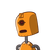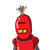# The distance between Chennai and Delhi is around 1800 km and on a map it isrepresented by 12 cm. Find the actual distance be

The distance between Chennai and Delhi is around 1800 km and on a map it is
represented by 12 cm. Find the actual distance between Chennai and Bangalore
represented on map as 3.5 cm.​

### 2 thoughts on “The distance between Chennai and Delhi is around 1800 km and on a map it is<br />represented by 12 cm. Find the actual distance be”

1.Step-by-step explanation:

1800/12=150

therefore 1 cm= 150 cm

3.5*150=525km

so distance between chennai and bangalore is 525km

2.Step-by-step explanation:

The distance between Chennai and Delhi =1800 km

on a map it is represented =12 cm

actual distance between Chennai and Bangalore represented on map 3.5 cm

=1800/12

$$\times$$

3.5

150 * 3.5

=525 Km